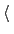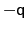Next: Linear and polynomial combinations Up: Collective variable components (basis Previous: Configuration keywords shared by   Contents   Index

Subsections

## Advanced usage and special considerations

### Periodic components.

The following components returns real numbers that lie in a periodic interval:
• dihedral: torsional angle between four groups;
• spinAngle: angle of rotation around a predefined axis in the best-fit from a set of reference coordinates.
In certain conditions, distanceZ can also be periodic, namely when periodic boundary conditions (PBCs) are defined in the simulation and distanceZ's axis is parallel to a unit cell vector.

The following keywords can be used within periodic components (and are illegal elsewhere):

• periodPeriod of the componentContext: distanceZ
Acceptable values: positive decimal
Default value: 0.0
Description: Setting this number enables the treatment of distanceZ as a periodic component: by default, distanceZ is not considered periodic. The keyword is supported, but irrelevant within dihedral or spinAngle, because their period is always 360 degrees.

• wrapAroundCenter of the wrapping interval for periodic variablesContext: distanceZ, dihedral or spinAngle
Acceptable values: decimal
Default value: 0.0
Description: By default, values of the periodic components are centered around zero, ranging fromto, whereis the period. Setting this number centers the interval around this value. This can be useful for convenience of output, or to set lowerWall and upperWall in an order that would not otherwise be allowed.
Internally, all differences between two values of a periodic colvar follow the minimum image convention: they are calculated based on the two periodic images that are closest to each other.

Note: linear or polynomial combinations of periodic components may become meaningless when components cross the periodic boundary. Use such combinations carefully: estimate the range of possible values of each component in a given simulation, and make use of wrapAround to limit this problem whenever possible.

### Non-scalar components.

When one of the following components are used, the defined colvar returns a value that is not a scalar number:
• distanceVec: 3-dimensional vector of the distance between two groups;
• distanceDir: 3-dimensional unit vector of the distance between two groups;
• orientation: 4-dimensional unit quaternion representing the best-fit rotation from a set of reference coordinates.
The distance between two 3-dimensional unit vectors is computed as the angle between them. The distance between two quaternions is computed as the angle between the two 4-dimensional unit vectors: because the orientation represented byis the same as the one represented by, distances between two quaternions are computed considering the closest of the two symmetric images.

Non-scalar components carry the following restrictions:

• Calculation of total forces (outputTotalForce option) is currently not implemented.
• Each colvar can only contain one non-scalar component.
• Binning on a grid (abf, histogram and metadynamics with useGrids enabled) is currently not implemented for colvars based on such components.

Note: while these restrictions apply to individual colvars based on non-scalar components, no limit is set to the number of scalar colvars. To compute multi-dimensional histograms and PMFs, use sets of scalar colvars of arbitrary size.

### Calculating total forces.

In addition to the restrictions due to the type of value computed (scalar or non-scalar), a final restriction can arise when calculating total force (outputTotalForce option or application of a abf bias). total forces are available currently only for the following components: distance, distanceZ, distanceXY, angle, dihedral, rmsd, eigenvector and gyration.Next: Linear and polynomial combinations Up: Collective variable components (basis Previous: Configuration keywords shared by   Contents   Index
vmd@ks.uiuc.edu